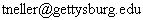CS 371 - Introduction to Artificial Intelligence Homework #5

Due: Part 1 is due Thursday 11/7 at the beginning of class.  The other parts are due Thursday 11/14.
Note: Work is to be done in pairs
1. Stochastic Simulation:  Implement stochastic simulation of a Bayesian network as described in Section 4.4.3 of "Probabilistic Reasoning in Intelligent Systems" by Judea Pearl.  Much convenient groundwork has been done for you.  In file bnSimulate.tar, you will find a collection of files that parse input and construct a Bayesian network for you.  You should only be concerned with two files: BNStochasticSimulation.java, which you modify, and BNNode.java, which you do not modify.  Within BNStochasticSimulation.java, there is a single method for you to implement: simulate.  Do not modify other parts of the code.
• Input Format:  To enter Table 1 of the above reference, you would create a file like sample.in:

• P(a) = {.20}
P(b|a) = {.20, .80}
P(c|a) = {.05, .20}
P(d|b,c) = {.05, .80, .80, .80}
P(e|c) = {.60, .80}
evidence
-d
e

The grammar for the input file is as follows:
<inputFile>  <--  <cptList> [ "evidence" <evidenceList>] <EOF>
<cptList>  <--  ( <cpt> )+
<cpt> <--  "P" "(" <varName> [ "|" <parentList> ] ")" "=" "{" <pvalueList> "}"
<parentList>  <--  <varName> ( "," <varName> )*
<pvalueList>  <--  <pvalue> ( "," <pvalue> )*
<evidenceList>  <--  <evidence> ( "," <evidence> )*
<evidence>  <--  "-" <varName> | <varName>
where <varName> is an alphanumeric variable name starting with a letter, and <pvalue> is a probability value.

CPT entries should be topologically sorted so that variables are not used as conditioning variables before their own CPT entry has been defined.  For example, if "P(b|a)  = ..." came before "P(a) = ..." above, the parser would complain.  Evidence is optional, but if there is no evidence, omit the keyword "evidence" as well.  Variable names are case sensitive.

Output Format:  Output format should mimic that of the sample output files.  For example, the output from the command
java BNStochasticSimulation 10000000 2000000 < sample.in > sample.out
would yield output of the form:
(Bayesian network information omitted.)
...
______________________________________________________________________
After iteration 2000000:
Variable, Average Conditional Probability, Fraction True
a, 0.09748785356892184, 0.097478
b, 0.09742460714035203, 0.097626
c, 0.031191558153934026, 0.031382
______________________________________________________________________
After iteration 4000000:
Variable, Average Conditional Probability, Fraction True
a, 0.09741916070759787, 0.0974705
b, 0.09741049285045494, 0.097506
c, 0.031176752524133284, 0.0313245
______________________________________________________________________
After iteration 6000000:
Variable, Average Conditional Probability, Fraction True
a, 0.09748330237820788, 0.09761716666666667
b, 0.0974821559496393, 0.09763166666666667
c, 0.03120110850466848, 0.0313455
______________________________________________________________________
After iteration 8000000:
Variable, Average Conditional Probability, Fraction True
a, 0.09740884107956631, 0.097467125
b, 0.09740182857956917, 0.097492875
c, 0.031173577904451428, 0.0312755
______________________________________________________________________
After iteration 10000000:
Variable, Average Conditional Probability, Fraction True
a, 0.09732317572895542, 0.097338
b, 0.09733498930038403, 0.0973159
c, 0.03114377427753685, 0.0312345

The first command line parameter is the number of iterations.  The second is the frequency of result reporting.

Note that for each non-evidence node we print both the average conditional probability and the fraction of iterations the variable has been assigned true.

As your first exercise, implement the stochastic simulation method such that your output is similar to that above.  For guidance, the exact conditional probability of variable a for the above problem is .0972762646.

2. Read Chapter 15.  Encode the Bayesian network of Figure 15.2 for input to the program above.  Include the input and output file (with no evidence) in your README file.  Use at least 1000000 iterations for these and other experiments.

3.
4. Focus on the latter part of section 15.2.  Use this Figure 15.2 problem to demonstrate d-separation.  There are three ways that evidence can "block" influence of one node on another.  Use your program to demonstrate each of the three cases.

5.
6. Describe what evidence and/or lack thereof is necessary for nodes b and c to be conditionally independent in the given Bayesian network (sample2.in).

7.
8. At the top of page 447, four different types of probabilistic inference are described.  For each type, use the given Bayesian Network (sample2.in) with and without different types of evidence and show such inference in action.  (Note that the values at the top of 447 in combination with your work in problem 2 provide helpful cases for testing problem 1.)

9. NOTE: In these latter problems, please put the final result of each output in your README file and comment on it.  It is not necessary (nor is it desirable) to have an enormous README file.  You need only supply your Bayesian network input file for problem 2 once. For each demonstration experiment, you need only describe the (1) evidence (or lack thereof) and (2) final results both before and after the introduction of new evidence.  Comment on each part!  Don't assume I can make sense of what you're doing amidst a sea of variables and numbers.

Todd Neller